# [python从入门到实践]绘图显示不出中文字

## 前言

（文末送读者福利）

``````import csv

import matplotlib.pyplot as plt

filename = 'sitka_weather_07-2018_simple.csv'
with open(filename) as f:
highs = []
high = int(row)
highs.append(high)

plt.style.use('seaborn')
fig,ax = plt.subplots()
ax.plot(highs,c='red')
ax.set_title("2018年7月每日最高温度",fontsize=24)
ax.set_xlabel('',fontsize = 16)
ax.set_ylabel("温度(F)",fontsize=16)
ax.tick_params(axis='both',which='major',labelsize=16)
````````````# 汉字字体，优先使用楷体，找不到则使用黑体
plt.rcParams['font.sans-serif'] = ['Kaitt', 'SimHei']

# 正常显示负号
plt.rcParams['axes.unicode_minus'] = False
plt.show()
``````# 零基础Python学习资源介绍

## 👉Python学习路线汇总👈

Python所有方向的技术点做的整理，形成各个领域的知识点汇总，它的用处就在于，你可以按照上面的知识点去找对应的学习资源，保证自己学得较为全面。（学习教程文末领取哈）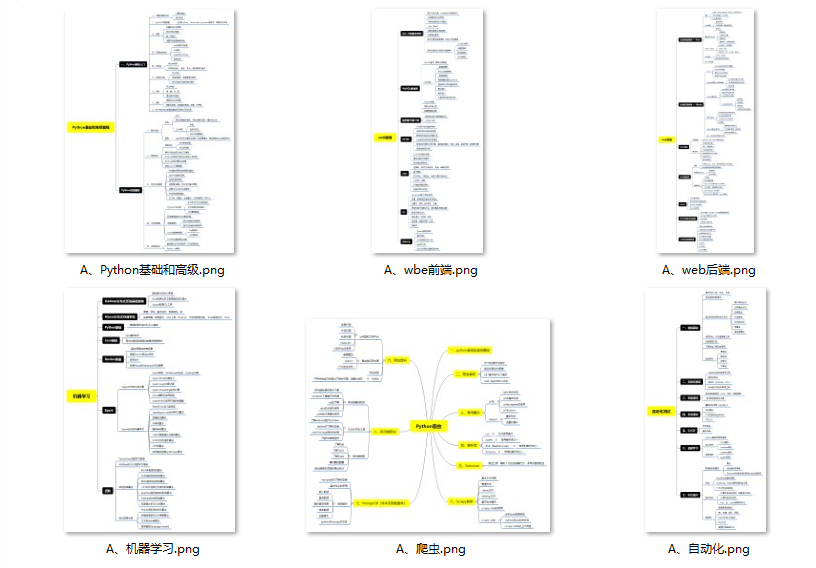## 👉Python必备开发工具👈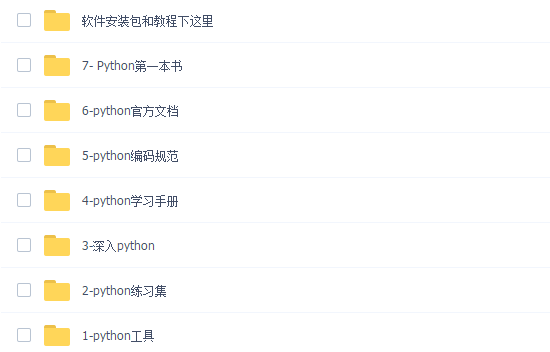## 👉Python学习视频600合集👈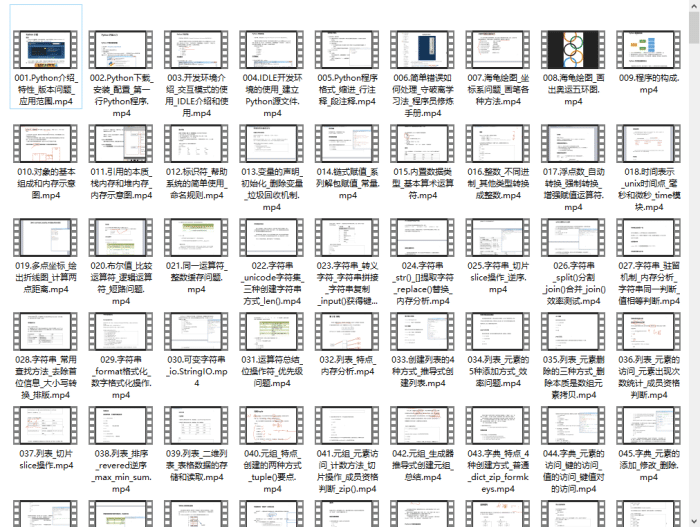## 👉实战案例👈## 👉面试刷题👈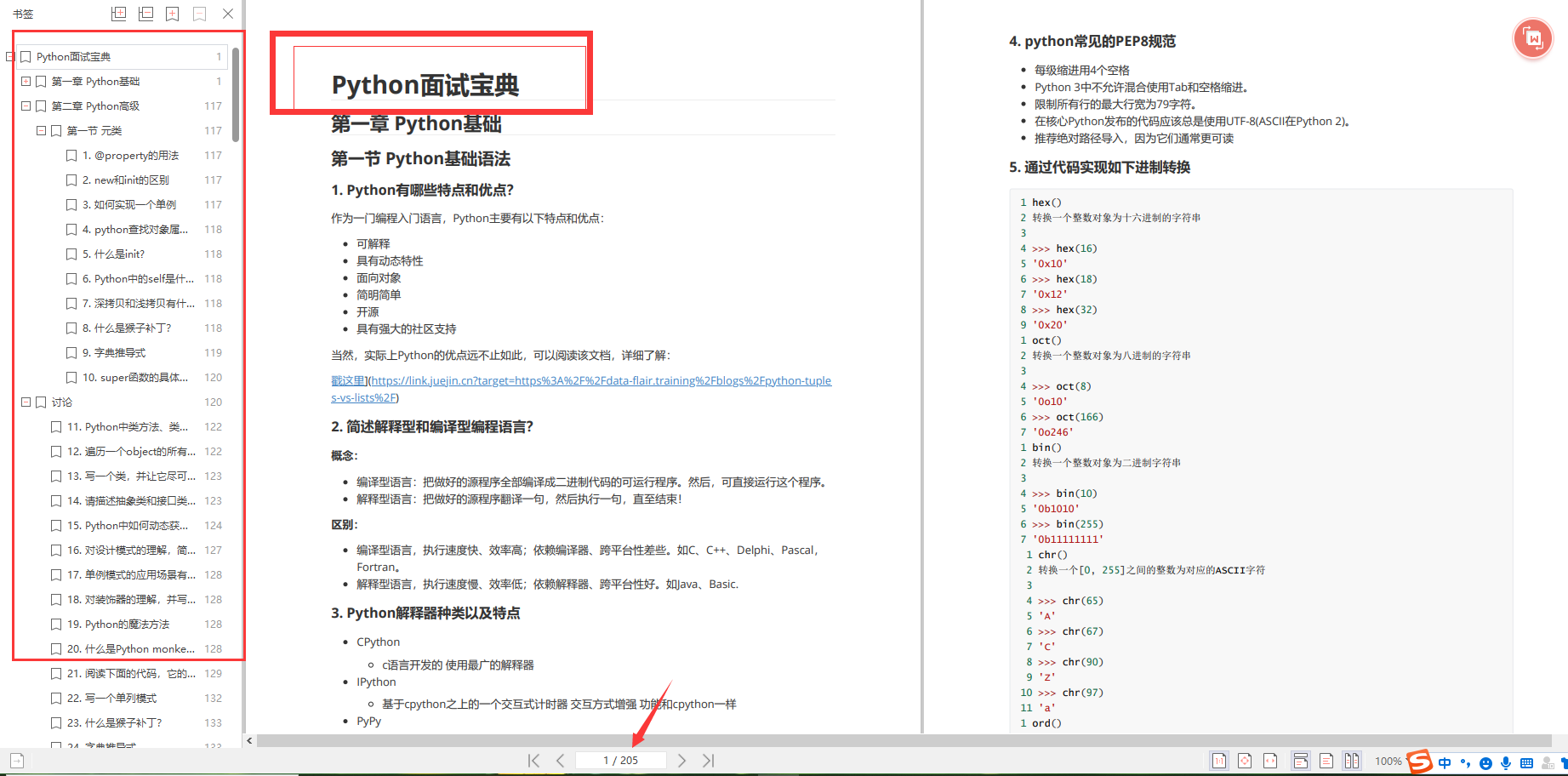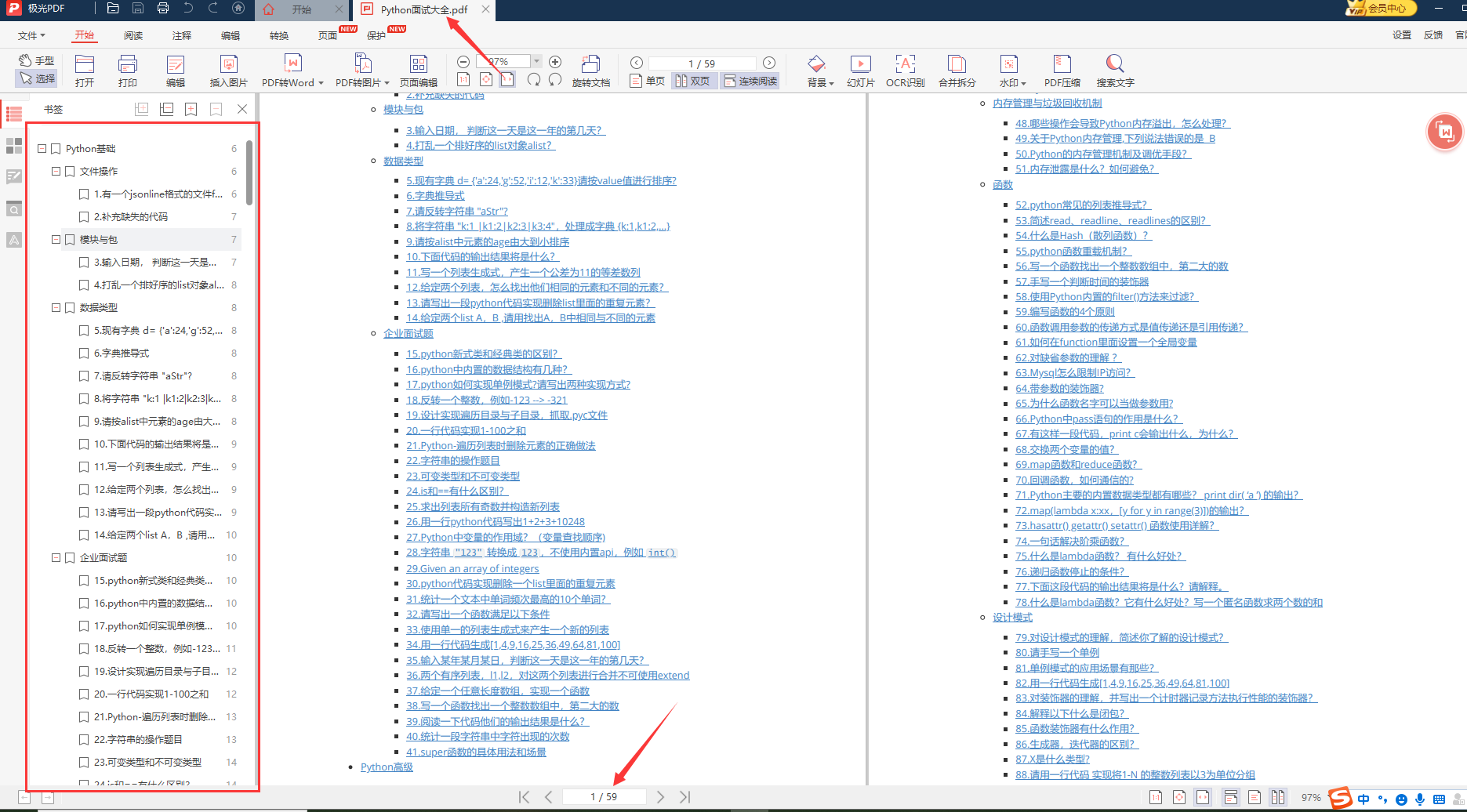# 资料领取# 好文推荐

THE END